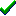# fg_3Dtexturemapobject()Win32DirectXFastgraph/Light

Prototype

void fg_3Dtexturemapobject (double *xyz_array, void *uv_array, int n);

Sub fg_3Dtexturemapobject (xyz_array() As Double, uv_array() As Any, ByVal n As Long)

procedure fg_3Dtexturemapobject (var xyz_array : double; var uv_array; n : integer);

Description

The fg_3Dtexturemapobject() function draws a linear or perspective texture-mapped polygon defined in 3D object space, with optional z-buffering and 3D clipping. Backface removal is performed unless drawing a z-buffered polygon. The polygon is drawn in 3D world space at the position and orientation specified in the most recent call to fg_3Dmoveobject() or fg_3Dsetobject().

Parameters

xyz_array is an array containing the 3D object space (x,y,z) coordinates for each polygon vertex. The first three xyz_array elements represent the (x,y,z) values at the polygon's first vertex, the next three xyz_array elements are for the second vertex, and so forth.

uv_array is an array containing the (u,v) texture map coordinates for each polygon vertex. The first two uv_array elements represent the (u,v) values at the polygon's first vertex, the next two uv_array elements are for the second vertex, and so forth. The fg_tmunits() function defines if uv_array contains integer or 32-bit floating point values.

n is the number of vertices in each of the above arrays.

Return value

none

Restrictions

If you attempt to fill a non-convex polygon with fg_3Dtexturemapobject(), only a part of it will be filled.

See also

Examples

Contents
Fastgraph Home Page

copyright 2001 Ted Gruber Software, Inc.